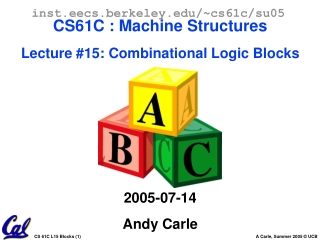DownloadDownload PresentationOutline

# Outline

Télécharger la présentation## Outline

- - - - - - - - - - - - - - - - - - - - - - - - - - - E N D - - - - - - - - - - - - - - - - - - - - - - - - - - -
##### Presentation Transcript

1. inst.eecs.berkeley.edu/~cs61c/su05CS61C : Machine StructuresLecture #15: Combinational Logic Blocks2005-07-14Andy Carle

2. Outline • CL Blocks • Latches & Flip Flops – A Closer Look

3. Review (1/3) • Use this table and techniques we learned to transform from 1 to another

4. (2/3): Circuit & Algebraic Simplification

5. (3/3):Laws of Boolean Algebra

6. CL Blocks • Let’s use our skills to build some CL blocks: • Multiplexer (mux) • Adder • ALU

7. N instances of 1-bit-wide mux

8. How do we build a 1-bit-wide mux?

9. 4-to-1 Multiplexor?

10. An Alternative Approach Hierarchically!

11. Arithmetic and Logic Unit • Most processors contain a logic block called “Arithmetic/Logic Unit” (ALU) • We’ll show you an easy one that does ADD, SUB, bitwise AND, bitwise OR

12. Our simple ALU

13. Truth-table, then determine canonical form, then minimize and implement as we’ve seen before Look at breaking the problem down into smaller pieces that we can cascade or hierarchically layer Adder/Subtracter Design -- how?

14. + + + N 1-bit adders  1 N-bit adder b0

18. # ± Whatop? What about overflow? • Consider a 2-bit signed # & overflow: • 10 = -2 + -2 or -1 • 11 = -1 + -2 only • 00 = 0 NOTHING! • 01 = 1 + 1 only • Highest adder • C1 = Carry-in = Cin, C2 = Carry-out = Cout • No Cout or Cin NO overflow! • Cin, and Cout NO overflow! • Cin, but no Cout A,B both > 0, overflow! • Cout, but no Cin A,B both < 0, overflow!

19. # ± What about overflow? • Consider a 2-bit signed # & overflow: 10 = -2 + -2 or -111 = -1 + -2 only00 = 0 NOTHING!01 = 1 + 1 only • Overflows when… • Cin, but no Cout A,B both > 0, overflow! • Cout, but no Cin A,B both < 0, overflow!

20. Extremely Clever Subtractor

21. Administrivia • We’re now halfway through the semester… yikes • HW 45 Due Monday • Proj2 coming… • Logisim!

22. State Circuits Overview • State circuits have feedback, e.g. • Output is function ofinputs + fed-back signals. • Feedback signals are the circuit's state. • What aspects of this circuit might cause complications? Combi-national Logic

23. A simpler state circuit: two inverters • When started up, it's internally stable. • Provide an or gate for coordination: • What's the result? 0 1! 0 1 0 1 0 1 0 1 0 1 0 0 1 How do we set to 0?

24. An R-S latch (cross-coupled NOR gates) A B NOR 0 0 1 0 1 0 1 0 0 1 1 0 • S means “set” (to 1),R means “reset” (to 0). • Adding Q’ gives standard RS-latch: _Q 0 0 1 0 0 1 1 0 0 1 0 1 0 1 1 0 Hold! Hold! 0 1 0 0 0 1 Truth table S R Q 0 0 hold (keep value) 0 1 0 1 0 1 1 1 unstable

25. An R-S latch (in detail) Truth table _S R Q Q Q(t+t) 0 0 0 1 0 hold 0 0 1 0 1 hold 0 1 0 1 0 reset 0 1 1 0 0 reset 1 0 0 1 1 set 1 0 1 0 1 set 1 1 0 x x unstable 1 1 1 x x unstable A B NOR 0 0 1 0 1 0 1 0 0 1 1 0

26. Controlling R-S latch with a clock • Can't change R and S while clock is active. • Clocked latches are called flip-flops. A B NOR 0 0 1 0 1 0 1 0 0 1 1 0

27. D flip-flop are what we really use • Inputs C (clock) and D. • When C is 1, latch open, output = D (even if it changes, “transparent latch”) • When C is 0, latch closed, output = stored value. C D AND 0 0 0 0 1 0 1 0 0 1 1 1

28. D flip-flop details • We don’t like transparent latches • We can build them so that the latch is only open for an instant, on the rising edge of a clock (as it goes from 01) D C Q Timing Diagram

29. Edge Detection • This is a “rising-edge D Flip-Flop” • When the CLK transitions from 0 to 1 (rising edge) … • Q  D; Qbar  not D • All other times: Q  Q; Qbar  Qbar A B O 0 0 1 0 1 1 1 0 1 1 1 1

30. Peer Instruction • Truth table for mux with 4 control signals has 24 rows • We could cascade N 1-bit shifters to make 1 N-bit shifter for sll, srl • If 1-bit adder delay is T, the N-bit adder delay would also be T

31. “And In conclusion…” • Use muxes to select among input • S input bits selects 2S inputs • Each input can be n-bits wide, indep of S • Implement muxes hierarchically • ALU can be implemented using a mux • Coupled with basic block elements • N-bit adder-subtractor done using N 1-bit adders with XOR gates on input • XOR serves as conditional inverter